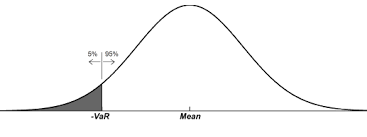策略無限

# 策略評量指標簡介

2019 年 3 月 27 日## Sharpe Ratio 夏普值

$$Sharpe=\frac{R_p-R_f}{\sigma_p}$$

$$R_p$$：投資商品的報酬率
$$R_f$$：無風險利率
$$\sigma_p$$：投資商品報酬率的標準差

## System Quality Number 系統品質指標

$$SQN=\sqrt{N}\cdot\frac{\mu_r}{\sigma_r}$$

$$N$$：交易次數
$$\mu_r$$：平均每筆損益
$$\sigma_r$$：每筆損益的標準差

System Quality Number （SQN）是系統品質指標，近年來也有許多投資者將此指標利用在策略品質的鑑定上，SQN 指標會在期望獲利越高、標準差越小、交易次數越多的情況下，整體的值越大。利用這個特性可以有效地衡量策略的獲利能力和穩定度。

## Return／Risk Ratio 風報比

$$RR=\frac{P}{MDD}$$

$$P$$：淨利
$$MDD$$：最大回撤（Max Drawdown）

Return／Risk Ratio（RR）是一個投資人必備的觀念，由 Victor Sperandeo 在「專業投機原理」上提出並應用，他也於交易市場獲得連續 18 年的長期正報酬，穩定一直都是交易理論的真正核心，風報比可說是判斷策略穩定度最好的基準。

## Value at Risk 風險值

$$Prob(X_T<-VaR)=\alpha$$

$$X_T$$：商品在持有 T 時間的損益
$$\alpha$$：信心水準【延伸閱讀】策略無限